## Figure Aesthetics or Overlays?

Tinkering with a new chart type over the weekend, I spotted something rather odd in in my F1 track history charts – what look to be outliers in the form of cars that hadn’t been lapped on that lap appearing behind the lap leader of the next lap, on track.If you count the number of cars on that leadlap, it’s also greater than the number of cars in the race on that lap.

How could that be? Cars being unlapped, perhaps, and so “appearing twice” on a particular leadlap – that is, recording two laptimes between consecutive passes of the start/finish line by the race leader?My fix for this was to add an “unlap” attribute that detects whether

```#Overplot unlaps

This groups by leadlap an car, and counts 1 for each occurrence. So if the `unlap` count is greater than 1, a car a has completed more than 1 lap in a given leadlap.

My first thought was to add this as an overprint on the original chart:

```#Overprint unlaps
g = g + geom_point(data = lapTimes[lapTimes['unlap']>1,],
```

This renders as follows:Whilst it works, as an approach it is inelegant, and had me up in the night pondering the use of overlays rather than aesthetics.

Because we can also view the fact that the car was on its second pass of the start/finish line for a given lead lap as a key property of the car and depict that directly via an aesthetic mapping of that property onto the symbol type:

```  g = g + geom_point(aes( x = trackdiff, y = leadlap,
pch= (unlap==1) ))+scale_shape_identity()```

This renders just a single mark on the chart, depicting the diff to the leader *as well as * the unlapping characteristic, rather than the two marks used previously, one for the diff, the second, overprinting, mark to depict the unlapping nature of that mark.So now I’m wondering – when would it make sense to use multiple marks by overprinting?

Here’s one example where I think it does make sense: where I pass an argument into the chart plotter to highlight a particular driver by infilling a marker with a symbol to identify that driver.

```#Drivers of interest passed in using construction: code=list(c("STR","+"),c("RAI","*"))
if (!is.na(code)){
for (t in code) {
g = g + geom_point(data = lapTimes[lapTimes['code'] == t, ],
aes(x = trackdiff, y = leadlap),
pch = t)
}
}```In this case, the + symbol is not a property of the car, it is an additional information attribute that I want to add to that car, but not the other cars. That is, it is a property of my interest, not a property of the car itself.

## Creating Simple Interactive Visualisations in R-Studio: Subsetting Data

Watching a fascinating Google Tech Talk by Hadley Wickham on The Future of Interactive Graphics in R – A Joint Visualization and UseR Meetup, I was reminded of the manipulate command provided in R-Studio that lets you create slider and dropdown widgets that in turn let you dynamically interact with R based visualisations, for example by setting data ranges or subsetting data.

Here are a couple of quick examples, one using the native plot command, the other using ggplot. In each case, I’m generating an interactive visualisation that lets me display as a line chart two user selected data series from a larger data set.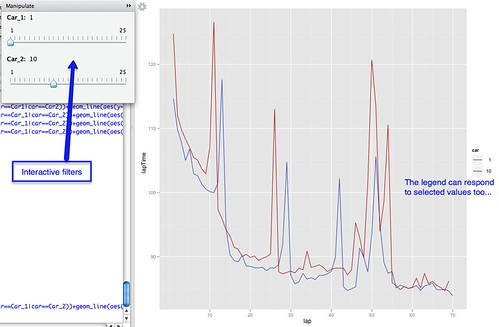Here’s a crude first attempt using plot:

View(hun_2011comprehensiveLapTimes)

library("manipulate")
h=un_2011comprehensiveLapTimes

manipulate(
plot(lapTime~lap,data=subset(h,car==cn1),type='l',col=car) +
lines(lapTime~lap,data=subset(h,car==cn2 ),col=car),
cn1=slider(1,25),cn2=slider(1,25)
)

This has the form manipulate(command1+command2, uiVar=slider(min,max)), so we see for example two R commands to plot the two separate lines, each of them filtered on a value set by the correpsonding slider variable.

Note that we plot the first line using plot, and the second line using lines.

The second approach uses ggplot within the manipulate context:

manipulate(
ggplot(subset(h,h\$car==Car_1|car==Car_2)) +
geom_line(aes(y=lapTime,x=lap,group=car,col=car)) +
Car_1=slider(1,25),Car_2=slider(1,25)
)

In this case, rather than explicitly adding additional line layers, we use the group setting to force the display of lines by group value. The initial ggplot command sets the context, and filters the complete set of timing data down to the timing data associated with at most two cars.

We can add a title to the plot using:

manipulate(
ggplot(subset(h,h\$car==Car_1|car==Car_2)) +
geom_line(aes(y=lapTime,x=lap,group=car,col=car)) +
opts(title=paste("F1 2011 Hungary: Laptimes for car",Car_1,'and car',Car_2)),
Car_1=slider(1,25),Car_2=slider(1,25)
)

My reading of the manipulate function is that if you make a change to one of the interactive components, the variable values are captured and then passed to the R command sequences, which then executes as normal. (I may be wrong in this assumption of course!) Which is to say: if you write a series of chained R commands, and can abstract out one or more variable values to the start of the sequence, then you can create corresponding interactive UI controls to set those variable values by placing the command series with the manipulate() context.

## Data Driven Story Discovery: Working Up a Multi-Layered Chart

How many different dimensions (or “columns” in a dataset where each row represents a different sample and each column a different measurement taken as part of that sample) can you plot on a chart?

Two are obvious: X and Y values, which are ideal for representing continuous numerical variables. If you’re plotting points, as in a scatterplot, the size and the colour of the point allow you to represent two further dimensions. Using different symbols to plot the points gives you another dimension. So we’re up to five, at least.

Whilst I was playing with ggplot over the weekend, I fell into this view over F1 timing data:It shows the range of positions held by each car over the course of the race (cars are identified by car number on the x-axis, 1 to 25 (there is no 13), and range of positions on the y-axis. The colour, uninterestingly, relates to car number.

If you follow any of the F1 blogs, you’ll quite often see references to “driver of the day” discussions (e.g. Who Was Your Driver of the Day). Which got me wondering…could a variant of the above chart provide a summary of the race as a whole that would, at a glance, suggest which drivers had “interesting” races?

For example, if a driver took on a wide range of race positions during the race, might this mean they had an exciting time of it? If every car retained the same couple of positions throughout the race, was it a procession?

Having generated the base chart using the ggplot web interface, I grabbed the code used to generate it and took it into RStudio.

What was lacking in the original view was an explicit statement about the position of each car at the end of the race. But we can add this using a point, if we know the final race position*:ggplot() + geom_step(aes(x=h\$car, y=h\$pos, group=h\$car)) + scale_x_discrete(limits = c('VET','WEB','HAM','BUT','ALO','MAS','SCH','ROS','HEI','PET','BAR','MAL','','SUT','RES','KOB','PER','BUE','ALG','KOV','TRU','RIC','LIU','GLO','AMB')) + xlab(NULL) + opts(axis.text.x=theme_text(angle=-90, hjust=0)) + geom_point(aes(x=k\$driverNum, y=k\$classification))

Let’s add it using a different coloured mark: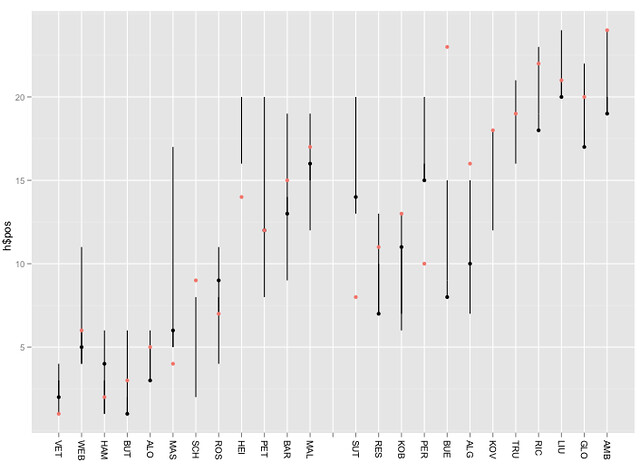+geom_point(aes(x=k\$driverNum, y=k\$grid,col='red'))

(Note that in the above example, the points are layered in the order they appear in the command line, with lower layers first. So if a car finishes (black dot) in the position it started (red dot), we will only see the red dot. If we make the lower layer dot slightly larger, we would then get a target effect in this case.)

The chart as we now have it shows where a driver started, where they finished and how many different race positions they found themselves in. So for example, we see BUE rapidly improved on his grid position at the start of the race and made progress through the race, but SUT went backwards from the off, as did PER.

Hmm… lots of race action seems to have happened during the first lap, but we’re not getting much sense of it… Maybe we need to add in the position of the car at the end of the first lap too. Unfortunately, the data I was using did not contain the actual grid/starting position (it just contains the positions at the end of each lap), but we can pull this data in from elsewhere… Using another dot to represent this piece of data might get confusing, so let’s use a line (set the symbol type using pch=3):+geom_point(aes(x=l\$car, y=l\$pos, pch=3))

Now we have a chart that shows the range of positions occupied by each car, their grid position, final race position and position at the end of the first lap Using different symbol types and colours we can distinguish between them. (For a presentation graphic, we also need a legend…) By using different sized symbols and layers, we could also display multiple dimensions at the same x, y co-ordinate.library("ggplot2")
h=hun_2011proximity
//in racestats, convert DNF etc to NA
k=hun_2011racestatsx
l=subset(h,lap==1)
ggplot() + geom_step(aes(x=h\$car, y=h\$pos, group=h\$car)) + scale_x_discrete(limits =c('VET','WEB','HAM','BUT','ALO','MAS','SCH','ROS','HEI','PET','BAR','MAL','','SUT','RES','KOB','PER','BUE','ALG','KOV','TRU','RIC','LIU','GLO','AMB')) + xlab(NULL) + opts(title="F1 2011 Hungary", axis.text.x=theme_text(angle=-90, hjust=0)) + geom_point(aes(x=l\$car, y=l\$pos, pch=3, size=2)) + geom_point(aes(x=k\$driverNum, y=k\$classification,size=2), label='Final') + geom_point(aes(x=k\$driverNum, y=k\$grid, col='red')) + ylab("Position")

A title can be added to the post using:

I still haven’t worked out how to do a caption that will display:
– [black dot] “Final Race Position”
– [red dot] “Grid Position”
– [tick mark] “End of lap 1”

Any ideas?

The y-axis gridline on the half is also misleading. To add gridlines for each position, add in +scale_y_discrete(breaks=1:24) or to label them as well +scale_y_discrete(breaks=1:24,limits=1:24)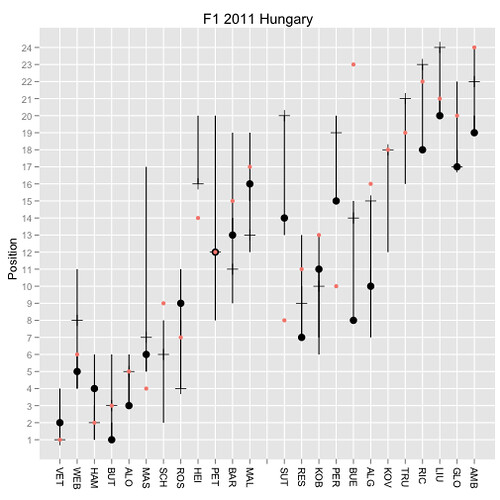So what do we learn from this? Layers can be handy and allow us to construct charts with different overlaid data sets. The order of the layers can make things easier or harder to read. Different symbol types work differently well with each other when overlaid. The same symbol shape in different sizes and appropriate layer ordering allows you to overlay points and still see them data. Symbol styles give the chart a grammar (I used circles for the start and end of the race positions, for example, and a line for the first lap position).

Lots of little things, in other words. But lots of little things that can allow us to add more to a chart and still keep it legible (arguably! I don’t claim to be a graphic designer, I’m just trying to find ways of communicating different dimensions within a data set).

* it strikes me that actually we could plot the final race position under the final classification. (On occasion, the race stewards penalise drivers so the classified result is different to the positions the cars ended the race in. Maybe we should make that eventuality evident?)

PS as @sidepodcast pointed out, I really should remove non-existent driver 13. I guess this means converting x-axis to a categorical one?

## Working Visually with the ggplot2 Web Interface…

We’re all familiar with line charts and pie charts, but how might we create new ways of visualising data. One way, is to play…

Over the weekend, I was doodling with an online, GUI driven hosted version of the ggplot graphing library for the statistical analysis language R: Jeroen C.L. Ooms (2010). yeroon.net/ggplot2: A web interface for the R package ggplot2

This service lets you upload a file and then using a handful of menu options create a wide variety of chart types. It also generates the corresponding ggplot/R commands so you can recreate the charts in your own R environment.

Because R is built for doing stats, ggplot has access to a powerful set of data transforming routines that can be applied to the data set you want to visualise. This includes being able to group data elements, plot different facets on different charts in a lattice style display, colour and size nodes according to a particular column and so on:

The data set I had was of the form related to (no surprises) F1 timing data. The columns included things like the driver name, car number, lap number and lap time, and stint number (each stint for a car is separated by a pitstop). Each row corresponded to data from a single lap for a single car. Which is to say, looking down the rows, we see driver names repeat (once for each lap they completed); or looking down the stint column, we can filter out rows that just correspond to the first or second stint for each driver. Which is to say: the data has structure, and can be viewed in a variety of ways.

So for example, in the following chart, we can plot for each driver (each separate graph, specified by treating the car number as a facet) their fuel corrected laptimes (y-axis) over the course of the race (lap number on x-axis). The colour of each point is determined by the pitstop column value which identifies whether a car has pitted on that lap: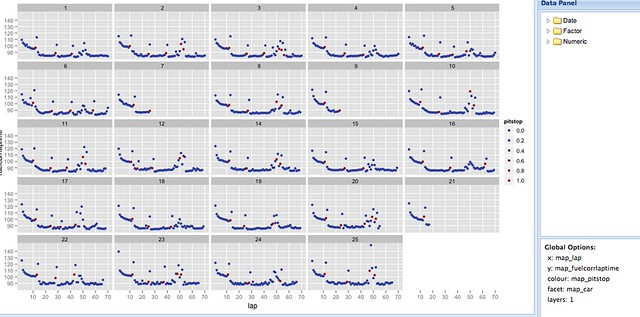The chart was created in six steps: one to upload the data, one to create a scatterplot layer and four menu selections (x, y, facet, colour).

As well as specifying facets, we can also group data rows to display them on the same faceted graph. Here, I group the data by car (so we will see a separate line for each car), and facet by stint: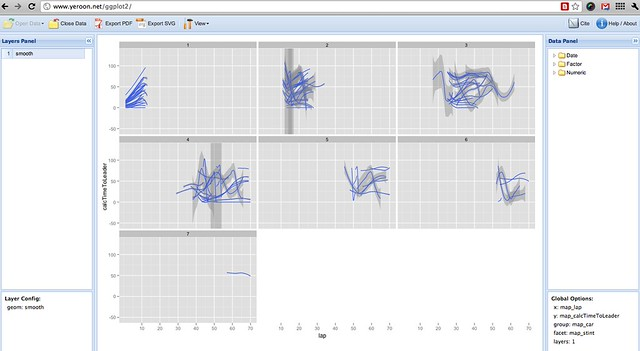Hopefully, you’ll see how it’s possible to explore a wide range of views over the data, cutting it up in all sorts of ways, quite easily. At a glance, we can get a view of different aspects of the whole data set, and start looking for surprising features that might be worth closer investigation. Because it’s quick to create different views over the data, we can quickly get an overall picture of how the data values are arranged.

There are issues of course: whilst the facet and group options provide one way of arranging the data, I don’t think there’s a way of filtering the data using the web interface (for example, to show the times by stint for cars 1 to 5). Adding support for filters (that is, data/subset operations) would make the web interface far more powerful as an interactive visual analysis tool, methinks, for example using the sort of dialogue used to specify filters in Gephi.

That said, I’ve now added Ooms’ ggplot web interface to the list of visualisation sites (along with IBM Many Eyes, for example), that I can use for getting a visual overview of a dataset quickly and easily. If you want to give it a go, you can find it at: http://www.yeroon.net/ggplot2/

Here’s a quick video overview if you need more convincing:

## Playing With R/ggplot2 Online (err, I think..?!)

Trying to get my head round what to talk about in another couple of presentations – an online viz tools presentation for the JISC activity data synthesis project tomorrow, and an OU workshop around the iChart eSTeEM project – I rediscovered an app that I’d completely forgotten about: an online R server that supports the plotting of charts using the ggplot library (err, I think?!): http://www.yeroon.net/ggplot2/

Example of how to use http://www.yeroon.net/ggplot2/

By the by, I have started trying to get my head round R using RStudio, but the online ggplot2 environment masks the stats commands and just focusses on helping you create quick charts. I randomly uploaded one of my F1 timing data files from the British Grand Prix, had a random click around, and in 8(?) clicks – from uploading the file, to rendering the chart – I’d managed to create this:What it shows is a scatterplot for each car showing the time on the current leader lap that the leader is ahead. When the plotted points drop from 100 or so seconds behind to just a few seconds behind, that car has been lapped.

What this chart shows (which I stumbled across just by playing with the environment) is a birds-eye view over the whole of the race, from each driver’s point of view. One thing I don’t make much use of is the colour dimension – or the size of each plotted point – but if tweak the input file to include the number of laps a car is behind the leader, their race position, the number of pitstops they’ve had, or their current tyre selection, I could easily view a couple more of these dimensions.

Where there’s a jump in the plotted points for a lap or two, if the step/break goes above the trend line (the gap to leader increases by 20s or so), the leader has lapped before the car. If the jump goes below the trend line (the gap to the leader has decreased), the leader has pitted before the car in question.

But that’s not really the point; what is the point is that here is a solution (and I think mirroring options are a possibility) for hosting within an institution an interactive chart generator. I also wonder to what extent it would be possible to extend the environment to detect single sign on credentials and allow a student to access a set of files related to a particular course, for example? Alternatively, it looks as if there is support for loading files in from Google Docs, so would it be possible to use this environment as a way of providing a graphing environment for data files stored (and maybe shared via a course) within a student’s Google Apps account?